## Example Questions

← Previous 1 3

### Example Question #1 : How To Find The Equation Of A Circle

In the standard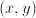coordinate plane, what is the radius and the center of the circle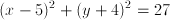?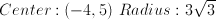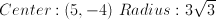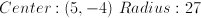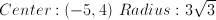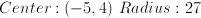Explanation:

When finding the center and radius of circle, the center is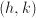and the radius is. Notice that they are not negative even though in the equation they have negative signs in front. This becomes important when dealing with real numbers. Also, notice the square of.

Our circle,has the same principles applied as the above principle, therefore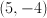is our center. Notice how the numbers signs have been switched. This is the case for all circles due to the negative in the base equation above.

To find the radius of a circle, you must take the number the equation is equal to and square root it. This is due to the square ofmentioned above. The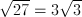. Use the least common multiples of 27 to find that three 3’s make up 27. Take two threes out as the square root of a number multiplied by itself is itself. This leaves one 3 under the radical. Therefore our radius is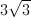.

Center:Radius:### Example Question #1 : How To Find The Equation Of A Circle

In the standard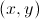coordinate plane, what is the area of the circle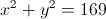?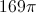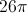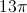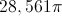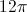Explanation:

The general equation of a circle is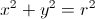.

According to the question,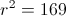. Thus,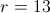.

The general equation for the area of a circle is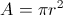.

When we plug in 13 for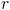, we get our area to equal.

### Example Question #1 : How To Find The Equation Of A Circle

A circle in the standard coordinate plane is tangent to the x-axis at (3,0) and tangent to the y-axis at (0,3). What is the equation of the circle?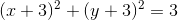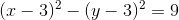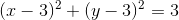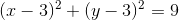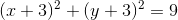Explanation:

The formula for the equation of a circle is (x – h)2+ (y – k)2 = r2, where (h, k) represents the coordinates of the center of the circle, and r represents the radius of the circle.

If a circle is tangent to the x-axis at (3,0), this means it touches the x-axis at that point. If a circle is tangent to the y-axis at (0,3), this means it touches the y-axis at that point. Given these two points, we can determine the center and the radius of the circle. The center of the circle must be equidistant from any of the points on the circumference. This means that both (0,3) and (3,0) are the same distance from the center. If we draw these points on a coodinate plane, it becomes apparent that the center of the circle must be (3,3). This point is exactly three units from each of the given points, indicating that the radius of the circle is 3.

When we input this information into the formula for a circle, we get (x – 3)+ (y – 3)= 9.

### Example Question #1 : How To Find The Equation Of A Circle

Which of the following gives the equation of a circle tangent to the line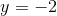with its center at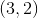?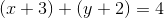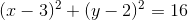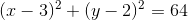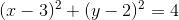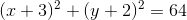Explanation:

The equation for a circle is (x - h)2 + (y - k)2 = r2, where (h,k) is the center of the circle and r is the radius.

We can eliminate (x+3) +(y+2) = 4 and (x+3)2 + (y+2)2 = 64. The first equation does not square the terms in parentheses, and the second refers to a center of (-3,-2) rather than (3,2).

Drawing the line y = -2 and a point at (3,2) for the center of the circle, we see that the only way the line could be tangent to the circle is if it touches the bottom-most part of the circle, directly under the center. The point of intersection will be (3,-2). From this, we can see that the distance from the center to this point of intersection is 4 units between (3,2) and (3,-2). This means the radius of the circle is 4.

Use the center point and the radius in the formula for a circle to find the final answer: (x - 3)2 + (y - 2)2 = 16

### Example Question #1 : How To Find The Equation Of A Circle

Find the equation of the circle with center coordinates of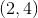and a radius of.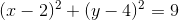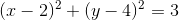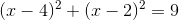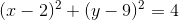Explanation:

The equation of a circle is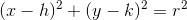The center isor, written another way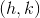. Substitutingforandfor, our formula becomes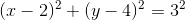Finally, the formula of the circle is### Example Question #1 : How To Find The Equation Of A Circle

On the xy plane, what is the area of a circle with the following equation: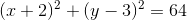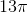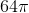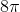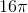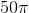Explanation:

The standard form equation of a circle is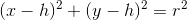, whereis the center of the circle andis equal to the radius. Thus, since we have the circle's standard form equation already given to us, we can ignoreand, since all we need is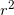.

The area of circle is equal to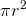, which is equal to.

### Example Question #1 : How To Find The Equation Of A Circle

A circle has a center at (5,5) and a radius of 2. If the format of the equation for the circle is (x+A)2+(y+B)2=C, what is C?

4

2

1

3

5

4

Explanation:

The circle has a center at (5,5) and a radius of 2. Therefore, the equation is (x+5)2+(y+5)2=22, or (x+5)2+(y+5)2=4.

### Example Question #1 : How To Find The Equation Of A Circle

If the center of a circle is at (0,4) and the diameter of the circle is 6, what is the equation of that circle?

x2 + y2 = 9

x2 + (y-4)2 = 36

(x-4)2 + y2 = 9

(x-4)2 + y2 = 36

x2 + (y-4)2 = 9

x2 + (y-4)2 = 9

Explanation:

The formula for the equation of a circle is:

(x-h) 2 + (y-k)2 = r2

Where (h,k) is the center of the circle.

h = 0 and k = 4

and diameter = 6 therefore radius = 3

(x-0) 2 + (y-4)2 = 32

x2 + (y-4)2 = 9

### Example Question #1 : How To Find The Equation Of A Circle

Circle A is given by the equation (x – 4)2 + (y + 3)2 = 29. Circle A is shifted up five units and left by six units. Then, its radius is doubled. What is the new equation for circle A?

(x – 10)2 + (y + 8)2 = 58

(x – 10)2 + (y + 8)2 = 116

(x + 2)2 + (y – 2)2 = 116

(x – 2)2 + (y + 2)2 = 58

(x + 2)2 + (y – 2)2 = 58

(x + 2)2 + (y – 2)2 = 116

Explanation:

The general equation of a circle is (x – h)2 + (y – k)2 = r2, where (h, k) represents the location of the circle's center, and r represents the length of its radius.

Circle A first has the equation of (x – 4)2 + (y + 3)2 = 29. This means that its center must be located at (4, –3), and its radius is √29.

We are then told that circle A is shifted up five units and then left by six units. This means that the y-coordinate of the center would increase by five, and the x-coordinate of the center would decrease by 6. Thus, the new center would be located at (4 – 6, –3 + 5), or (–2, 2).

We are then told that the radius of circle A is doubled, which means its new radius is 2√29.

Now, that we have circle A's new center and radius, we can write its general equation using (x – h)2 + (y – k)2 = r2.

(x – (–2))2 + (y – 2)2 = (2√29)2 = 22(√29)2 = 4(29) = 116.

(x + 2)2 + (y – 2)2 = 116.

The answer is (x + 2)2 + (y – 2)2 = 116.

### Example Question #1 : How To Find The Equation Of A Circle

Which of the following equations describes all the points (x, y) in a coordinate plane that are five units away from the point (–3, 6)?

(x – 3)2 + (y + 6)2 = 25

(x + 3)2 + (y – 6)2 = 25

y + 6 = 5 – (x – 3)2

(x – 3)2 + (y + 6)2 = 5

(x – 3)2 – (y + 6)2 = 25

(x + 3)2 + (y – 6)2 = 25

Explanation:

We are trying to find an equation for all of the points that are the same distance (5 units) from (–3, 6). The locus of all points equidistant from a single point is a circle. In other words, we need to find an equation of a circle. The center of the circle will be (–3, 6), and the radius, which is the distance from (–3,6), will be 5.

The standard form of a circle is given below:

(x – h)2 + (y – k)2 = r2, where the center is located at (h, k) and r is the length of the radius.

In this case, h will be –3, k will be 6, and r will be 5.

(x – (–3))2 + (y – 6)2 = 52

(x + 3)2 + (y – 6)2 = 25

The answer is (x + 3)2 + (y – 6)2 = 25.

← Previous 1 3

### All ACT Math Resources